Next: Standardized Database Performance Up: Applying the Model Previous: Applying the Model

## Expectation and Arg Max

We shall now describe a technique for computing the argmax for a mixture model using the bounding techniques introduced earlier. Traditionally, the mean of each Gaussian is evaluated to determine its probability and the best mean is returned as the arg max. This is not always the correct solution and we derive a technique with which the true arg max can be computed.

Observe the Gaussian mixture model shown in Figure 7.12 (what we get after training the model and observing a new covariate input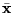. It is composed of 4 Gaussians which are shown alone with dotted lines. We would like to solve for the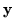which maximizes the expression. One may be tempted to just try settingto the prototype of each Gaussian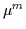and checking to see which one of these is the best. The 4 prototypes points are shown as circles on the pdf and none of them is the maximum. This is due to interaction between the individual models and the maximum could, for instance, lie between two Gaussians. Therefore, this is usually not the arg max. In addition, we show the expectation value on the curve (the average) by a * symbol and also note that it is not maximal.

We will consider the prototypes as seeds and try optimizing the probability from there. Thus, we should converge to the global maximum of probability after investigating all possible attractors. For MGaussians, there are M attractor basins and these usually have non-zero mutual interaction. Thus, assume we are at a current operating point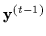(seeded at one of the Gaussian means or prototypes). We wish to find a better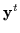using an iterative approach. Equivalently, we can maximize the logarithm of the difference in probabilities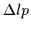as in Equation 7.34.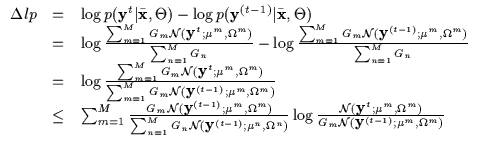(7.34)

Applying Jensen's inequality yields a bound which is very similar to our previous Q-function in the EM algorithm. Effectively, we propose solving arg maximization using an EM framework with multiple seeds (a total of M seeds for M Gaussians). In fact, the application of Jensen's inequality above is the E-step. The M-step is obtained by taking derivatives with respect toof the bound and setting them to 0. We obtain the update equation for theas in Equation 7.35. This E and the M steps are iterated andmonotonically converges to a local maximum. For each of the 4 seeds, we show the convergence on the mixture model in Figure 7.13(a) and the monotonic increase in Figure 7.13(b). Given that only a handful of iterations are needed and only M Gaussians are being used, the complete arg maximization process we implemented requires only several milliseconds to find the bestoccupying a vector space of dimensionality greater than 100.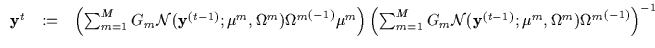(7.35)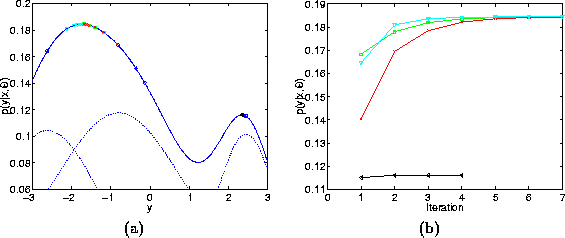If time is a critical factor, we can use standard techniques to compute a mere expectation of the mixture model which is given in Equation 7.36. This solution, often has lower probability than the arg max.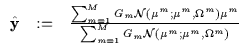(7.36)Next: Standardized Database Performance Up: Applying the Model Previous: Applying the Model
Tony Jebara
1999-09-15